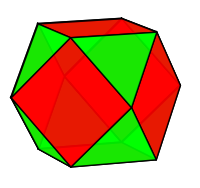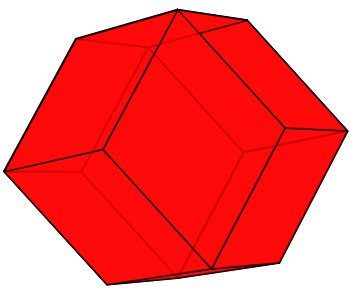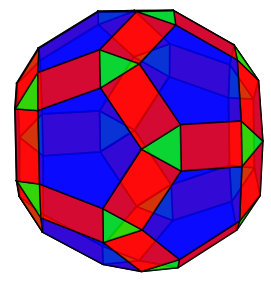# Edge-Ploding Polyhedra

Geometry Level 1

Let $P$ be a polyhedron that is the convex hull of the vertices $v_1, v_2, \ldots , v_n$ and with edges $e_1, e_2, \ldots , e_m$ where each edge is defined to be the set of points on the line that passes through some two distinct vertices $v_i, v_j$.

We define the Edge-Plosion of $P$, $E!( P )$, to be the convex hull of the mid-points of each edge of $P$, where $P$ is a cube.

Which of these is an image of $E!(E!(P))$?

(A):(B):(C):(D):×